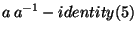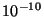IdentifiantMot de passe
Mot de passe oublié ?
17.2 Python Interface

# 17.2 Python Interface

All examples in this section assume that you performed a

```from numarray import *
import numarray.linear_algebra as la
```

 cholesky_decomposition( a)
This function returns a lower triangular matrix L which, when multiplied by its transpose yields the original matrix `a`; `a` must be square, Hermitian, and positive definite. L is often referred to as the Cholesky lower-triangular square-root of `a`.

 determinant( a)
This function returns the determinant of the square matrix `a`.
```>>> print a
[[ 1  2]
[ 3 15]]
>>> print la.determinant(a)
9.0
```

 eigenvalues( a)
This function returns the eigenvalues of the square matrix `a`.
```>>> print a
[[ 1.    0.    0.    0.  ]
[ 0.    2.    0.    0.01]
[ 0.    0.    5.    0.  ]
[ 0.    0.01  0.    2.5 ]]
>>> print la.eigenvalues(a)
[ 2.50019992  1.99980008  1.          5.        ]
```

 eigenvectors( a)
This function returns both the eigenvalues and the eigenvectors, the latter as a two-dimensional array (i.e. a sequence of vectors).
```>>> print a
[[ 1.    0.    0.    0.  ]
[ 0.    2.    0.    0.01]
[ 0.    0.    5.    0.  ]
[ 0.    0.01  0.    2.5 ]]
>>> eval, evec = la.eigenvectors(a)
>>> print eval  # same as eigenvalues()
[ 2.50019992  1.99980008  1.          5.        ]
>>> print transpose(evec)
[[ 0.          0.          1.          0.        ]
[ 0.01998801  0.99980022  0.          0.        ]
[ 0.          0.          0.          1.        ]
[ 0.99980022 -0.01998801  0.          0.        ]]
```

 generalized_inverse( a, rcond=1e-10)
This function returns the generalized inverse (also known as pseudo-inverse or Moore-Penrose-inverse) of the matrix `a`. It has numerous applications related to linear equations and least-squares problems.
```>>> ainv = la.generalized_inverse(a)
>>> print array_str(innerproduct(a,ainv),suppress_small=1,precision=8)
[[ 1.  0.  0.  0.]
[ 0.  1.  0. -0.]
[ 0.  0.  1.  0.]
[ 0. -0.  0.  1.]]
```

 Heigenvalues( a)
returns the (real positive) eigenvalues of the square, Hermitian positive definite matrix a.

 Heigenvectors( a)
returns both the (real positive) eigenvalues and the eigenvectors of a square, Hermitian positive definite matrix a. The eigenvectors are returned in an (orthornormal) two-dimensional matrix.

 inverse( a)
This function returns the inverse of the specified matrix a which must be square and non-singular. To within floating point precision, it should always be true that ```matrixmultiply(a, inverse(a)) == identity(len(a))```. To test this claim, one can do e.g.:
```>>> a = reshape(arange(25.0), (5,5)) + identity(5)
>>> print a
[[  1.   1.   2.   3.   4.]
[  5.   7.   7.   8.   9.]
[ 10.  11.  13.  13.  14.]
[ 15.  16.  17.  19.  19.]
[ 20.  21.  22.  23.  25.]]
>>> inv_a = la.inverse(a)
>>> print inv_a
[[ 0.20634921 -0.52380952 -0.25396825  0.01587302  0.28571429]
[-0.5026455   0.63492063 -0.22751323 -0.08994709  0.04761905]
[-0.21164021 -0.20634921  0.7989418  -0.1957672  -0.19047619]
[ 0.07936508 -0.04761905 -0.17460317  0.6984127  -0.42857143]
[ 0.37037037  0.11111111 -0.14814815 -0.40740741  0.33333333]]
```
Verify the inverse by printing the largest absolute element of:
```>>> print "Inversion error:", maximum.reduce(fabs(ravel(dot(a, inv_a)-identity(5))))
Inversion error: 8.18789480661e-16
```

 linear_least_squares( a, b, rcond=1e-10)
This function returns the least-squares solution of an overdetermined system of linear equations. An optional third argument indicates the cutoff for the range of singular values (defaults to). There are four return values: the least-squares solution itself, the sum of the squared residuals (i.e. the quantity minimized by the solution), the rank of the matrix a, and the singular values of a in descending order.

 solve_linear_equations( a, b)
This function solves a system of linear equations with a square non-singular matrix a and a right-hand-side vector b. Several right-hand-side vectors can be treated simultaneously by making b a two-dimensional array (i.e. a sequence of vectors). The function inverse(a) calculates the inverse of the square non-singular matrix a by calling solve_linear_equations(a, b) with a suitable b.

 singular_value_decomposition( a, full_matrices=0)
This function returns three arrays V, S, and WT whose matrix product is the original matrix a. V and WT are unitary matrices (rank-2 arrays), whereas S is the vector (rank-1 array) of diagonal elements of the singular-value matrix. This function is mainly used to check whether (and in what way) a matrix is ill-conditioned.

Send comments to the NumArray community.